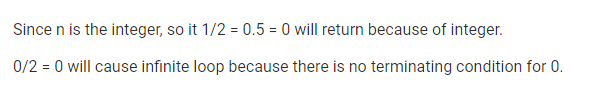# Gate CS-2019 Question Paper With Solutions

Q. 35 Consider the following C program:

``void` `convert(``int` `n) { ` `  ``if` `(n < 0) ` `    ``printf``(“ % d”, n); ` `  ``else` `{ ` `    ``convert(n / 2); ` `    ``printf``(“ % d”, n % 2); ` `  ``} ` `} ``

Which one of the following will happen when the function convert is called with any positive integer n as argument?

(A) It will print the binary representation of n in the reverse order and terminate.

(B) It will print the binary representation of n but will not terminate

(C) It will not print anything and will not terminate.

(D) It will print the binary representation of n and terminate.